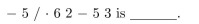# [Solved] The Value of the Arithmetic Expression Whose Preﬁx Representation Is

Question 6

## The value of the arithmetic expression whose preﬁx representation is10+ million students use Quizplus to study and prepare for their homework, quizzes and exams through 20m+ questions in 300k quizzes.

### Mathematics

Explore our library and get Discrete Mathematics Homework Help with various study sets and a huge amount of quizzes and questions

142

Study sets

2.1K

Quizzes

239.4K

Questions

Upload material to get free accessInvite a friend and get free accessSubscribe and get an instant access